Search IntMath
Close

450+ Math Lessons written by Math Professors and Teachers

5 Million+ Students Helped Each Year

1200+ Articles Written by Math Educators and Enthusiasts

Simplifying and Teaching Math for Over 23 Years

# Conic sections - summary

This is a summary of the first 5 topics in this chapter: straight line, circle, parabola, ellipse and hyperbola.

Don't miss the 3D interactive graph, where you can explore these conic sections by slicing a double cone.

## Straight Line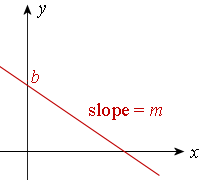Slope-intercept Form

The equation of a line with slope m and y-intercept b is given by:

y = mx + b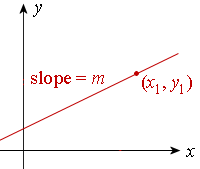Point-slope form

The equation of a line passing through a point (x1, y1) with slope m:

y y1 = m(xx1)

General Form of a Straight Line

Ax + By + C = 0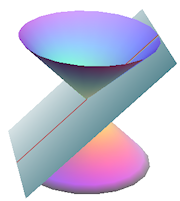Conic Section

If we slice the double cone by a plane just touching one edge of the double cone, the intersection is a straight line, as shown.

For background and examples, see Straight Line.

## Circle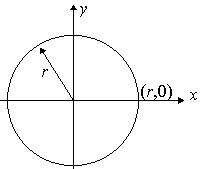The circle with centre (0, 0) and radius r has the equation:

x2 + y2 = r2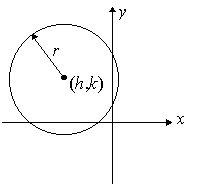The circle with centre (h, k) and radius r has the equation:

(xh)2 + (yk)2 = r2

General Form of the Circle

An equation which can be written in the following form (with constants D, E, F) represents a circle:

x2 + y2 + Dx + Ey + F = 0

Formal Definition
A circle is the locus of points that are equidistant from a fixed point (the center).

Conic Section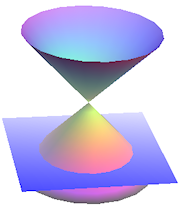If we slice one of the cones with a plane at right angles to the axis of the cone, the shape formed is a circle.

For background and examples, see Circle.

## Parabola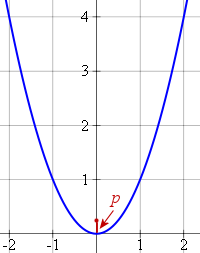Parabola with Vertical Axis

A parabola with focal distance p has equation:

x2 = 4py

In more familiar form, with "y = " on the left, we can write this as:

y=x^2/(4p)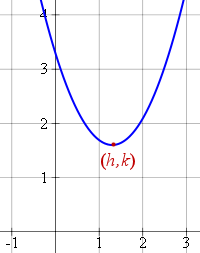If the axis of a parabola is vertical, and the vertex is at (h, k), we have

(xh)2 = 4p(yk)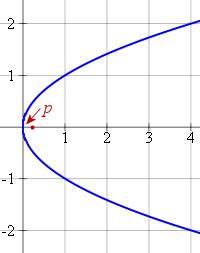Parabola with Horizontal Axis

In this case, we have the relation:

y2 = 4px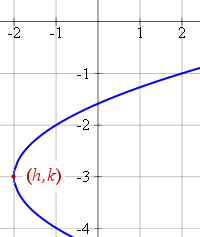If the axis of a parabola is horizontal, and the vertex is at (h, k), the equation becomes

(yk)2 = 4p(xh)

Formal Definition

A parabola is the locus of points that are equidistant from a point (the focus) and a line (the directrix).

Conic Section

If we slice a cone parallel to the slant edge of the cone, the resulting shape is a parabola, as shown.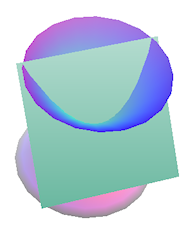For background and examples, see Parabola.

## Ellipse

Horizontal Major Axis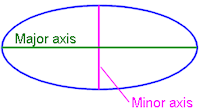The equation for an ellipse with a horizontal major axis and center (0,0) is given by:

x^2/a^2+y^2/b^2=1

The foci (plural of 'focus') of the ellipse (with horizontal major axis) are at (-c,0) and (c,0), where c is given by:

c=sqrt(a^2-b^2

The vertices of an ellipse are at (-a, 0) and (a, 0).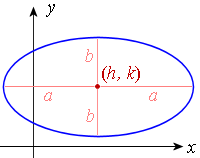An ellipse with horizontal major axis and with center at (h, k) is given by:

((x-h)^2)/a^2+((y-k)^2)/b^2=1

Vertical Major Axis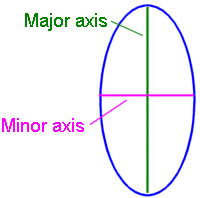If the major axis is vertical, then the formula becomes:

x^2/b^2+y^2/a^2=1

We always choose our a and b such that a > b.

Formal Definition

An ellipse is the locus of points whereby the sum of the distances from 2 fixed points (the foci) is constant.

Conic Section

When we slice one of the cones at an angle to the sides of the cone, we get an ellipse, as seen in the view from the top (at right).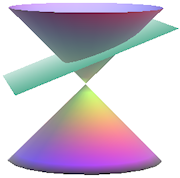For background and examples, see Ellipse.

## Hyperbola

North-south Opening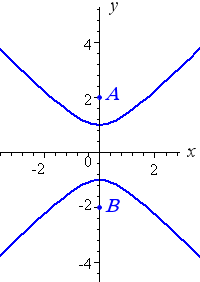For a north-south opening hyperbola:

y^2/a^2-x^2/b^2=1

The slopes of the asymptotes are given by:

+-a/b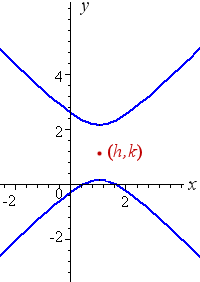For a "north-south" opening hyperbola with centre (h, k), we have:

((y-k)^2)/a^2-((x-h)^2)/b^2=1

East-west Opening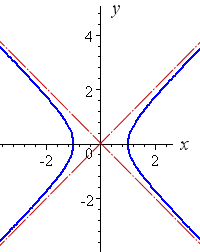For an east-west opening hyperbola:

x^2/a^2-y^2/b^2=1

The slopes of the asymptotes are given by:

+-b/a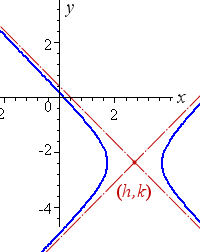For an "east-west" opening hyperbola with centre (h, k), we have:

((x-h)^2)/a^2-((y-k)^2)/b^2=1

Formal Definition

A hyperbola is the locus of points where the difference in the distance to two fixed foci is constant.

General Form of a Hyperbola

Ax^2 + Bxy + Cy^2 + Dx + Ey + F  = 0

(such that B^2>4AC)

Conic Section

When we slice our double cone such that the plane passes througn both cones, we get a hyperbola, as shown.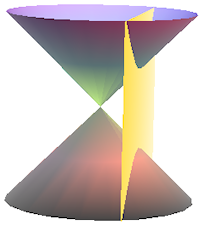For background and examples, see Hyperbola.

Also, don't miss the 3D interactive graph, where you can explore these conic sections by slicing a double cone.

## Problem SolverThis tool combines the power of mathematical computation engine that excels at solving mathematical formulas with the power of GPT large language models to parse and generate natural language. This creates math problem solver thats more accurate than ChatGPT, more flexible than a calculator, and faster answers than a human tutor. Learn More.